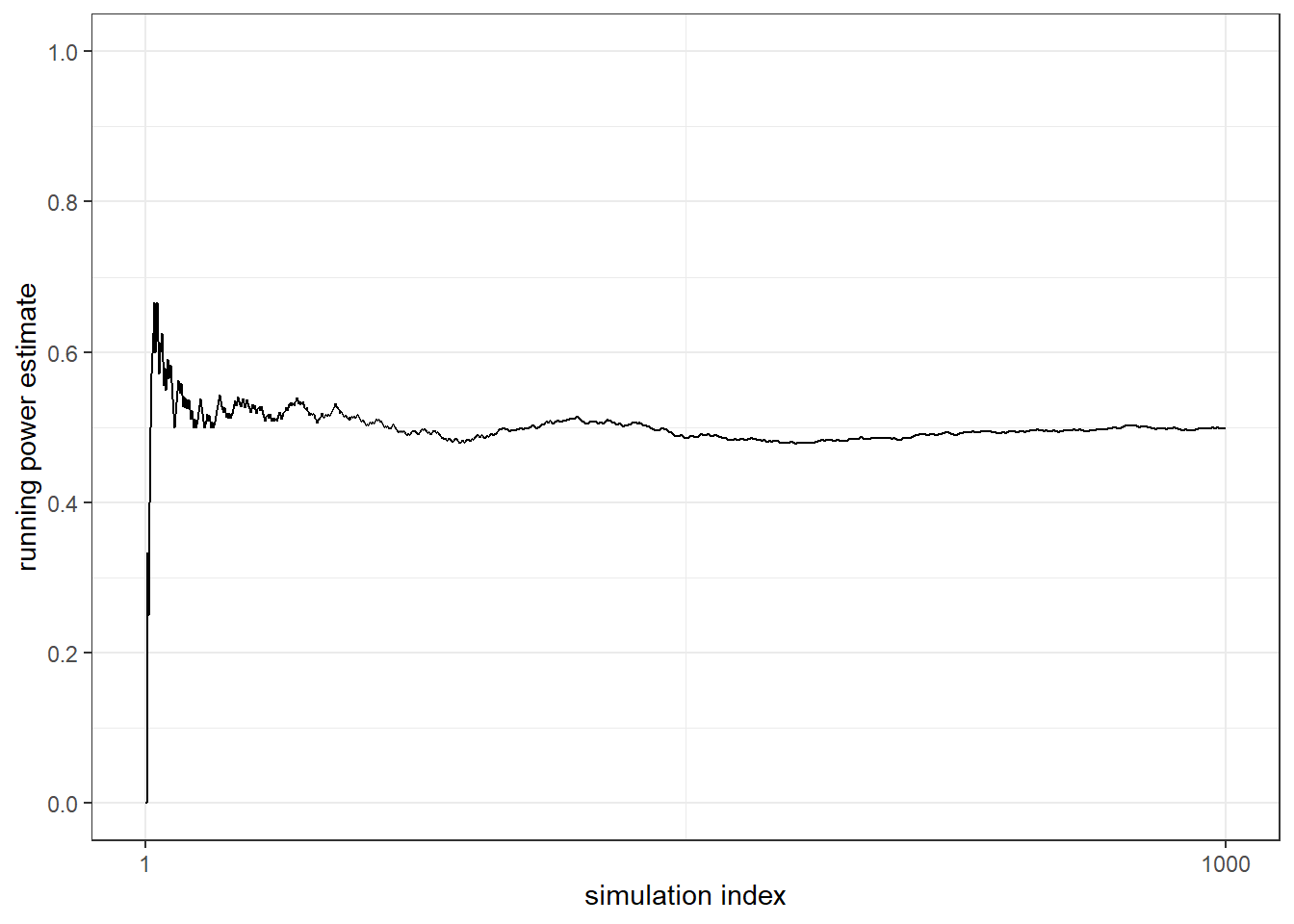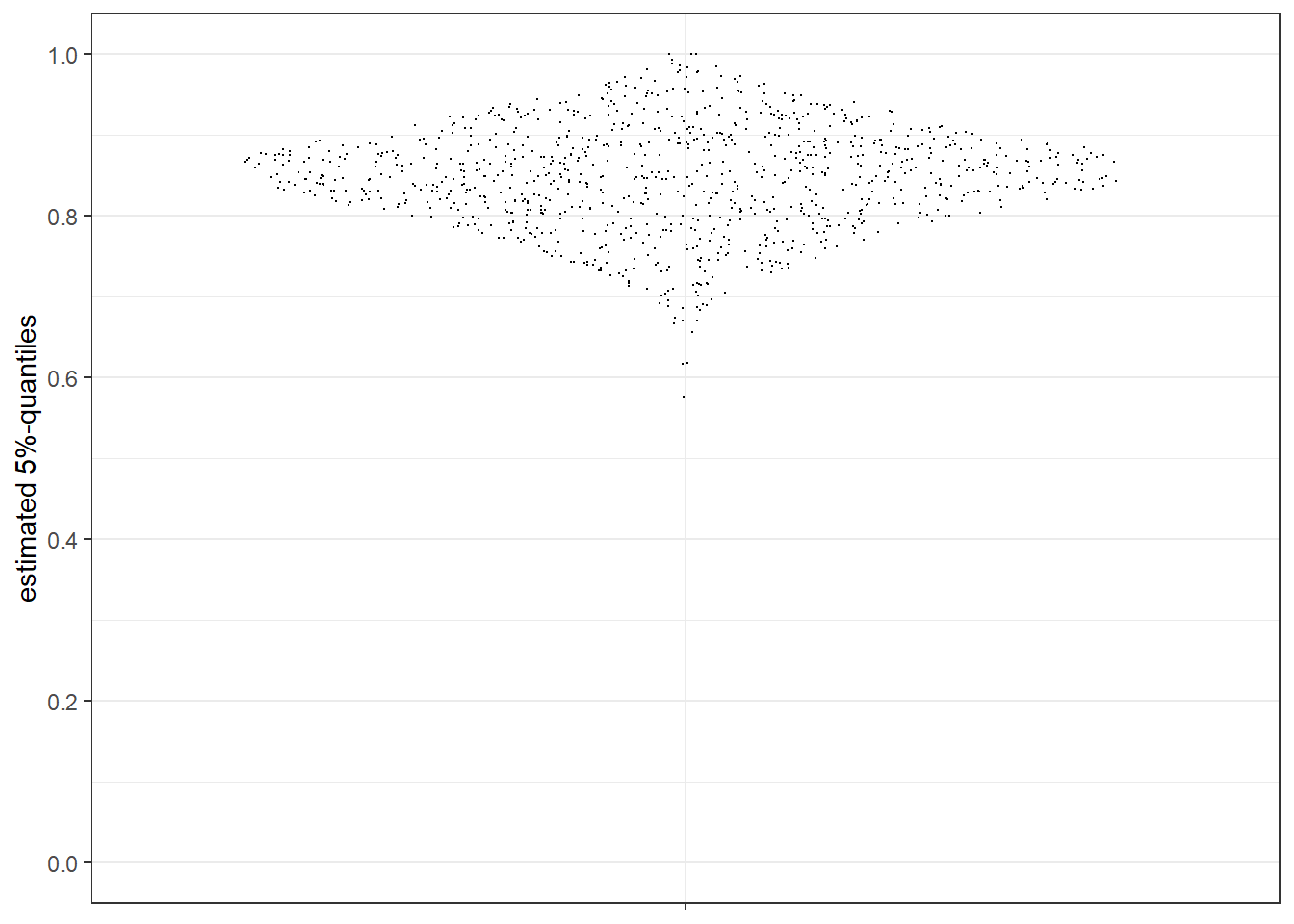## Introduction

The R package qcapower allows you to estimate power with regard to the consistency of a term generated in a Qualitative Comparative Analysis (QCA). Power is defined in the usual way as the probability of rejecting the null hypothesis when it is false. This vignette introduces the package, its in-built functions and what you can do with the functions’ output. The package includes four functions:

• qcapower(): Estimates power with regard to the consistency of a term
• qp_run_plot(): A plot of the running power estimates
• qp_quant_plot(): A plot of the 5%-percentiles of the permuted distributions
• qp_get_n(): Estimates the number of cases needed for achieving a target level of power

All these functions have a term as its subject. In a set-relational context, a term can be anything of the following: A single condition (individually sufficient or INUS), a conjunction, or a disjunction of conditions and conjunctions.

At the moment, the package estimates power for sufficient set relationships and fuzzy sets. In principle, it does not make a difference whether we are interested in sufficiency or necessity. For both types of set relations, the consistency measure is used to distinguish consistent from inconsistent set relations. However, the difference rests in how consistency is calculated and the package currently only implements the consistency formula for consistency.

## Estimating power

The qcapower() function can be used to estimate power given information about the other parameters that influence power. The code in brackets refers to the parameters in the qcapower() function. * cases: The number of cases (n) * null_hypo: The null hypothesis (H0)
* alt_hypo: The alternative hypothesis (H1)
* alpha: The level of significance alpha (default is 0.05)

The first parameters do not have default values and must be specified by the user. alpha is set to 0.05 by default.

In fuzzy-set QCA (fsQCA), determining the number of cases is easy because it is the total number of cases in an analysis. The null hypothesis determines the consistency value that separates consistent from inconsistent terms. When it comes to the assignment of outcome values to truth table rows when setting up the truth table for minimization, you might choose 0.85 as the value separating rows that are assigned a “1” (consistency is equal to or above 0.85) from those that receive a “0”. The null hypothesis would then cover the value of 0.849 (when we measure set memberships up to the third decimal place). The alternative hypothesis covers the consistency value that you expect a term to have, in fact. For power analysis, the consistency value of H1 must be larger than of H0 because we expect a term to be consistent.

You need to specify four more parameters for estimating power because the function implements permutation-based simulations.
* sims: The number of simulations (default is 1000)
* perms: The number of permutations per simulations (default is 10000)
* cons_threshold: A tolerance parameter for deriving simulated data with the consistenty level fixed with H1 (default is 0.01)
* set_seed: The reproducibility parameter for the simulations (default is 135, for no specific reason)

The role of the simulations and permutations is explained in the manuscript on which this package is based. The tolerance parameter cons_threshold is part of the package because of how it creates a hypothetical dataset with the consistency level specified by alt_hypo. The package first draws a random sample of n cases (cases) and calculates the consistency score. If it is smaller than the value of alt_hypo, one inconsistent case is picked at random from the set of all inconsistent cases. This case randomly receives a new set membership in the outcome that is higher than the previous outcome membership because it will increase the overall consistency of the dataset. If the consistency score of the dataset is above the target level, we follow the opposite procedure: A case is sampled for which membership in the outcome is larger than membership in the term and a lower outcome value is then reassigned to the case and consistency is calculated again.

These processes are repeated until the consistency score of the dataset achieves the target value of alt_hypo. Because we do not constrain fuzzy-set memberships to two decimal places, it is unlikely that the iterative process does exactly meet the target score. For this reason, we introduce a tolerance parameter telling the qcapower() function when the actual consistency value is sufficiently close to the intended value and the iteration can be stopped. If one wants to exactly hit the target consistency level, one only has to set cons_threshold to 0.

If you want to leave the default parameters as they are, you can, for example, estimate power for H1=1, H0=0.85 and n=15 with one line. The execution of qcapower() can take some time, depending on the settings for cases, perms and sims. We load simulation results into the memory that one would get from the first line in the following code block.

## power_example <- qcapower(cases = 15, alt_hypo = 1, null_hypo = 0.85)
head(qcapower_vign)
##   power  powercum alt_hypo null_hypo cases     quant
## 1 0.499 0.0000000        1      0.85    15 0.8104265
## 2 0.499 0.0000000        1      0.85    15 0.8457524
## 3 0.499 0.3333333        1      0.85    15 0.8763145
## 4 0.499 0.2500000        1      0.85    15 0.8248283
## 5 0.499 0.4000000        1      0.85    15 0.8935880
## 6 0.499 0.5000000        1      0.85    15 0.8664958

The output is a dataframe with as many rows as simulations. In addition to the parameters cases, alt_hypo and null_hypo, it contains three columns:

• power: The power estimate. The entry is the same in each row because it is the final power estimate for the entire dataset.
• powercum: The cumulative power estimate. It gives you the estimate up to the given row. The cumulative estimate of the last row equals the final power estimate.
• quant: The 5%-quantile of the permuted distribution underlying a row (see qp_quant_plot() below). This tells one whether the specified value for H0 is in the left tail of the permuted distribution.

If you are only interested in the power estimate and want a single number as the output, you might take a shortcut by running something like:

mean(qcapower(cases = 10, alt_hypo = 1, null_hypo = 0.8, sims = 10, perms = 1000)$power) qcapower(cases = 10, alt_hypo = 1, null_hypo = 0.8, sims = 10, perms = 1000)$power 

### Checking the number of simulations

The power estimate should be reliable in the sense that it should only change marginally if you add 10 or 50 additional simulations. The cumulative power plot is a visual means for checking whether sims is sufficiently large. This can be displayed with qp_run_plot(). You can add a title to the figure by specifying title = T.

qp_run_plot(qcapower_vign)Fig. 1: Plot of running power estimate

In this example, the estimate is in the range of 0.5 after about 300 simulations and stabilizes in this range. Setting sims to 1000 therefore seems appropiate. If you prefer a different visualization style, you can create your own plot by working with the powercum column of your dataset. The plot is made with ggplot2 and a gg object that could also be customized in the usual ways.

### Checking the dispersion of estimates

The dispersion of the permuted distributions is useful to look at when interpreting a power estimate. With a small number of cases, the width and location of the distributions can vary widely. Although I’d say that the power estimate is not invalid, I recommend checking the distribution of 5%-quantiles of each permuted distribution to understand how much underlying variation you have in the simulation. The more dispersed the 5%-quantiles are, the more careful you should be in attaching strong claims to a power estimate.

The function qp_quant_plot() plots the quantiles in a sina plot using the ggforce package (see on Github). A sina plot combines a dot plot with a violin plot by arranging the dots in the shape that a violin plot would take. The plot is a gg object and can be edited in the usual ways.

qp_quant_plot(qcapower_vign)Fig. 2: Plot of 5%-quantiles

A single plot of 5%-quantiles is not easy to interpret without comparing to another plot using a different number of cases. Even without such a comparison, however, one could infer here that the distribution is wide. The body of quantiles ranges from 1 to about 0.65 with the miminum being below 0.6.

## Estimating the number of cases

The function qp_cases() allows you to estimate how many cases you need to achieve a target level of power. To avoid running a simulation every time one wants an estimate of n, this function draws on an underlying dataset with estimates of n for different values of H1 and H0. The values of H1 and H0 are limited to the range of 0.75 to 0.95 in steps of 0.05; the possible values for H1 are 0.85 and 1. The number of cases ranges from 2 to 100 for each pair of H0 and H1 with H1 being larger than H0. Each combination of H0 and H1 was simulated each with 5000 simulations each with 50000 permutations. (In the future, we might expand the range of H1 values and implement a more fine-grained grid of values.)

qp_cases(0.9, null_hypo = 0.80, alt_hypo = 1)
##  33

The function qp_cases_brute() allows you to estimate how many cases you need to achieve a target level of power. The function input requires the desired level of power (power_target) and all parameters known from qcapower() except cases. The function runs simulations and iteratively searches for the number of cases yielding a power estimate that is sufficiently close to the target level. (see above on the closeness of the target level and the estimated level).

The simulation has to start with a given number of cases (start_value). The default is 20. The larger the difference between start value and the needed number of cases, the longer the simulation runs. max_value is the maximum number of cases that the function should work with. A reason to specify max_value could be that one knows that there are only n cases in the population and there is no point in estimating power for a higher number. The default is 50.

Note: Running the function may take some time with a high number of simulations and permutations.

# not run
qp_cases_brute(power_target = 0.9, null_hypo = 0.80, alt_hypo = 1)

## Packages used in this vignette and references

• base (R Core Team 2019)
• ggforce (Lin Pedersen 2019)
• ggplot2 (Wickham 2016)
• grateful (Rodriguez-Sanchez 2018)
• qcapower (Rohlfing and Doering, n.d.)
• usethis (Wickham and Bryan 2019)
• devtools (Wickham, Hester, and Chang 2019)

Pedersen, Thomas Lin. 2019. ggforce: Accelerating ‘ggplot2’. R package version 0.3.1.
https://CRAN.R-project.org/package=ggforce

R Core Team. 2019. R: A Language and Environment for Statistical Computing.
Vienna, Austria: R Foundation for Statistical Computing. https://www.R-project.org/.

Rodriguez-Sanchez, Francisco. 2018. grateful: Facilitate Citation of R Packages.
https://github.com/Pakillo/grateful.

Rohlfing, Ingo, and Holger Doering. n.d.
qcapower: Estimate Power in QCA and the Number of Cases Needed to Achieve a Target Level.
https://github.com/ingorohlfing/qcapower.

Wickham, Hadley. 2016. ggplot2: Elegant Graphics for Data Analysis. Springer-Verlag: New York.

Wickham, Hadley, and Jennifer Bryan. 2019. usethis: Automate Package and Project Setup. https://CRAN.R-project.org/package=usethis.

Wickham, Hadley, Jim Hester, and Winston Chang. 2019.
devtools: Tools to Make Developing R Packages Easier.
https://CRAN.R-project.org/package=devtools.